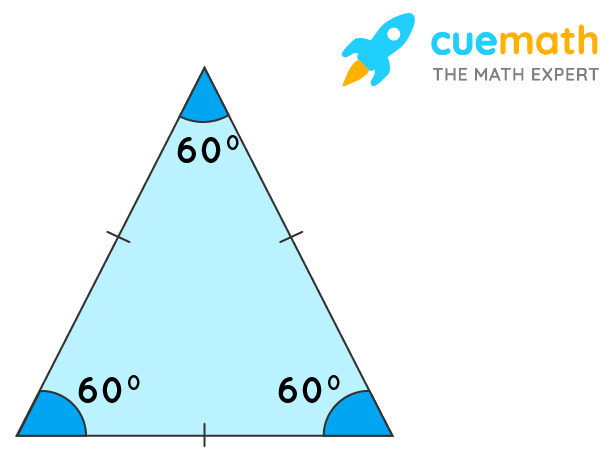# What Does Equilateral Triangle Look Like

## Question:  What does an equilateral triangle look like?

It is similar to other triangles accept all three sides are equal.

## Answer: Equilateral triangle is a 3-sided polygon whose all 3 sides are equal.

Let us understand the concept with an image.

## Explanation

An equilateral triangle is shown below.